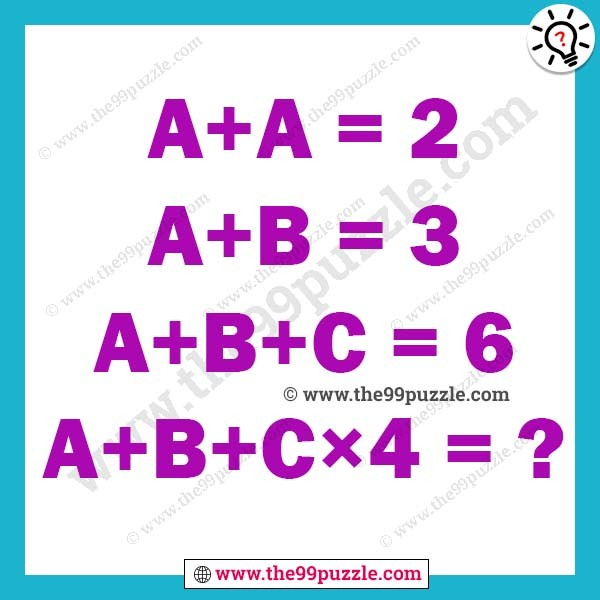# Brain teaser letter puzzles with answer – Puzz142

Can you solve the brain teaser letter puzzles? Here you will find many logical reasoning missing letter puzzles for students. If you are good at puzzles you can find the value of these brain teaser letter puzzles.

A+A=2

A+B=3

A+B+C=6

A+B+C×4=?###### Explanation:

A to Z  are respectively assign the numbers 1 to 26

A=1

B=2

C=3

A+A=1+1=2

A+B=1+2=3

A+B+C=1+2+3=6

A+B+C×4=1+2+3×4=15